PHY294H - Lecture 9

Recommended problems for Lecture 9: 25.7, 25.16, 25.18
Recommended reading for Lecture 10: pp 676-689

A distribution of point charges

Since superposition holds for the electric field, it also holds for the electric potential. If we have a set of charges
. The electric field at position
, which is at vector distance
from chargeis given by a vector superposition of the electric fields due to each of the charges. In a similar way, the electric potential due to this distribution of charges is given by,
(1)

The nice thing about this superposition is that it is a scalar. Furthermore, it is possible to find the electric field from the potential, as we now demonstrate.

Finding the electric field from the potential

It is easier to find the potential due to a set of point charges than it is to find the electric field directly. It would therefore be very nice if we could find the electric field from the potential. This is actually quite straightforward. The electric field is given by,
(2)

However we also know that the
can be written as,(3)

So we find,
(4)

This is written more succinctly as,
(5)

where
is the gradient operator. Its form depends on the co-ordinate system we choose. For the moment we only need cartesion co-ordinates, where the gradient is given by,
(6)

Another way to visualize the relation between the electric potential and the electric potential is to draw equipotential surfaces. On an equipotential surface, the electric potential is a constant. If the electric potential is a constant, then the electric field along the equipotential surface is zero. This means that the electric field is perpendicular to the equipotential surface. This gives us another way of visualizing electric field lines as well.

A point charge

The electric field is given by,
(7)

Thus,
(8)

The electric potential due to a dipole

Consider a dipole lying on the x-axis, with the positive charge atand the negative charge at
. The charges have magnitude
. A point in the x-y plane is defined by its radial distance to the origin,, and by an angle
between the directionand the x-axis. We want to find the electric potential due to the dipole as a function,
. The potential is,(9)

where,
(10)

and(11)

Using these expressions, we rewrite Eq. (8) as,
(12)

At long distances where
, we can use the expansions,
(13)

This yields,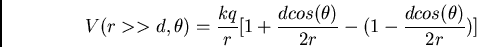(14)
(15)

where
is the magnitude of the dipole moment and we have used. The electric field is given by,(16)

which implies that,
(17)
(18)
(19)

This should be compared with the result we calculated for the electric field at
,andThe electric potential energy of a charge distribution

The potential energy of a chargeat positionis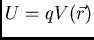. A different question is: What is the potential energy of a distribution of charges, that is, what is the potential energy stored in a distribution of charges. The potential energy stored in a distribution of charges is equal to the work done in setting up the distribution of charges, provided there is no dissipation and no kinetic energy is generated. To set up a distribution of chargesat positions, we need to bring each of the charges in from infinity and place it at its allocated position. The work required to place the first charge is zero (no other charges are there yet). The work required to place the second charge is
, where
is the electric potential at position
due to charge.
. The work required to place charge 3 at its position is equal to
, and so on, once all of the
charges are in position, we have,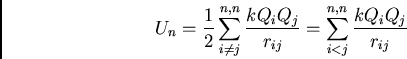(20)

In these expressions each pair interaction is counted once and the total potential energy is the sum of the potential energies of all pairs.